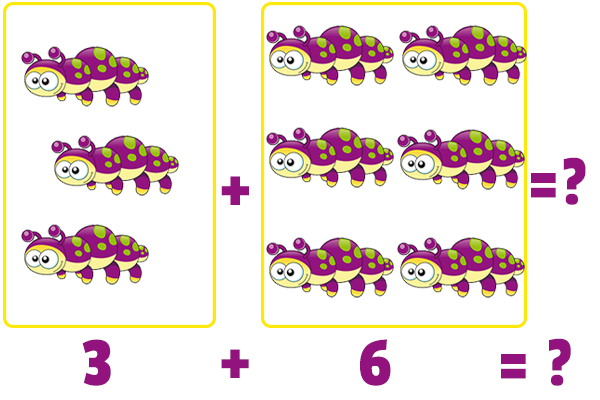# Addition Up to 10 Animals

Description: Add numbers up to ten with a fun animal themed math exercise.

Tags: Kindergarten, Preschool, Addition, CCSS.MATH.CONTENT.K.OA.A.1, Kindergarten Math Problems

Question 1 (Short Answer, 10 points)

What is 3 + 5 = ?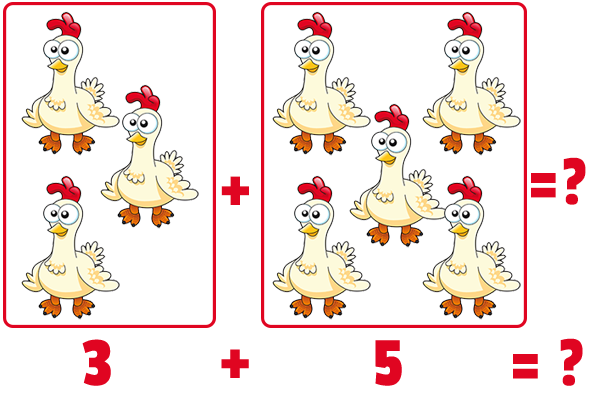Question 2 (Short Answer, 10 points)

What is 0 + 1 = ?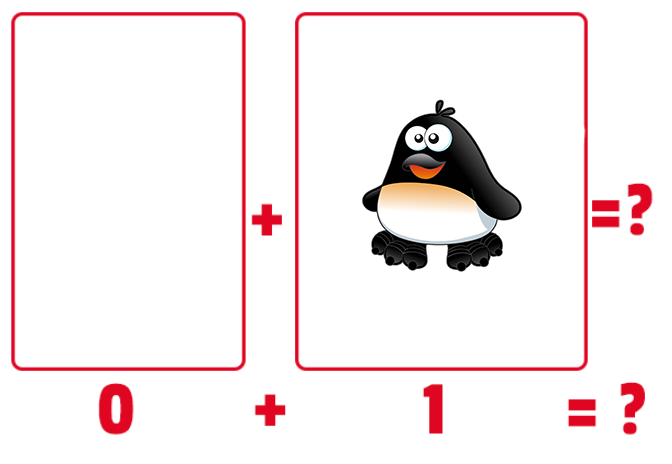Question 3 (Multiple Choice, 10 points)

What is 2 + 4 = ?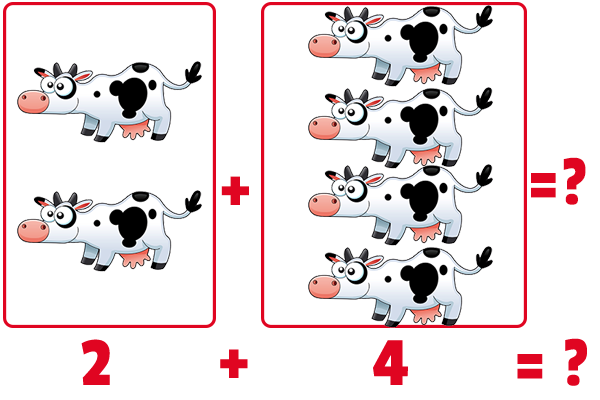Question 4 (Multiple Choice, 10 points)

What is 7 + 2 = ?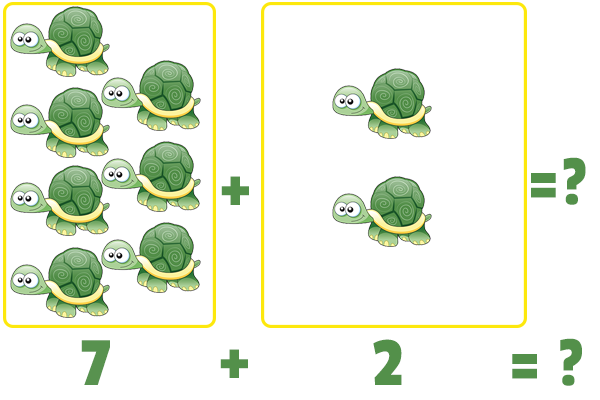Question 5 (Short Answer, 10 points)

What is 3 + 6 = ?